## Dynamics, Geometry, and Randomness

### Mathematica Examples

• Fractal Curves via Iteration (Mathematica 4.2 notebook, ~750k)
Samples created with this notebook: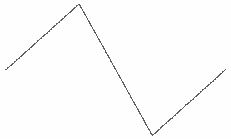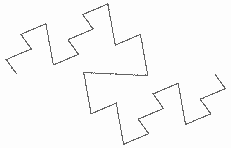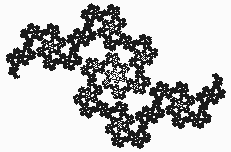### Mathematica Hints

• After opening a mathematica notebook, you need to re-evaluate each input cell (a section marked by a blue bracket at the right) by selecting it and pressing Shift-Enter. If you don't do this, mathematica won't know about the functions and objects defined in the notebook.
Example:
• Mathematica knows the definition of f(x) if you evaluate the cells in order: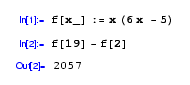• But if you don't evaluate the first cell, mathematica doesn't know the definition of the function: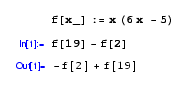• Mathematica can save notebook files in HTML format for viewing on the web, or for printing if you are having trouble with other methods. From the File menu, select Save As Special... and then HTML. Make sure you give a filename that ends with ".html". Mathematica will create that file and a subdirectory called "Images", which will store the graphics from the notebook (e.g. plots, fancy mathematical notation).